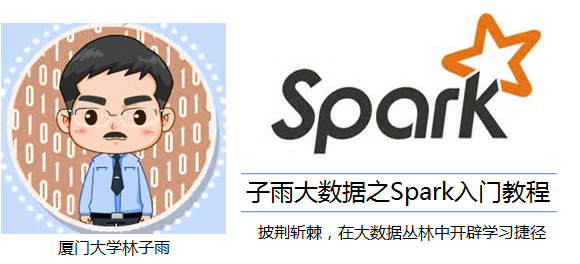﻿

# Scala:for循环

【版权声明】博客内容由厦门大学数据库实验室拥有版权，未经允许，请勿转载！
[返回Spark教程首页]

## for循环

Scala中的for循环语句格式如下：

``````for (变量<-表达式) 语句块
``````

``````for (i <- 1 to 5) println(i)
``````

``````1
2
3
4
5
``````

``````for (i <- 1 to 5 by 2) println(i)
``````

``````1
3
5
``````

``````for (i <- 1 to 5 if i%2==0) println(i)
``````

``````2
4
``````

Scala也支持“多个生成器”的情形，可以用分号把它们隔开，比如：

``````for (i <- 1 to 5; j <- 1 to 3) println(i*j)
``````

``````1
2
3
2
4
6
3
6
9
4
8
12
5
10
15
``````

``````for (i <- 1 to 5 if i%2==0; j <- 1 to 3 if j!=i) println(i*j)
``````

``````2
6
4
8
12
``````

## for推导式

``````scala> for (i <- 1 to 5 if i%2==0) yield i
res3: scala.collection.immutable.IndexedSeq[Int] = Vector(2, 4)
``````